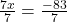## When the distributive property is used to solve the equation 10(x+8) =3x-3 what is the next step? pls help i will mark u brainli

Question

When the distributive property is used to solve the equation 10(x+8) =3x-3 what is the next step?

pls help i will mark u brainliest

in progress 0
2 weeks 2021-08-30T03:29:10+00:00 1 Answers 0 views 0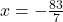Step-by-step explanation:

use distributive property: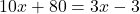you have to move all the terms with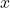to the left side of the equation:

subtract 3x from both sides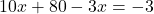combine like terms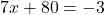subtract 80 from both sides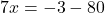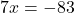divide each term by 7